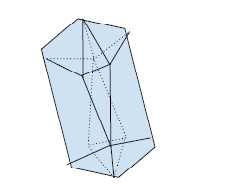# Program to find the Area and Volume of Icosahedron in C++

In this problem, we are given a value that denotes the side of an icosahedron. Our task is to create a program to find the Area and Volume of Icosahedron in C++.

Icosahedron is a regular 30 sided polyhedron. It has 20 equilateral triangles of the same side. There are only 12 vertices of this polyhedron.Dashed lines are for the edges that are behind the visible surface.

Let’s take an example to understand the problem,

## Input

a = 4

Program to illustrate the working of our solution,

## Example

Live Demo

#include <iostream>
using namespace std;
float calcIcoSArea(float a) {
return (8.660 * a * a);
}
float calcIcoVolume(float a) {
return (2.1817 * a * a * a);
}
int main(){
float a = 7;
cout<<"The length of side of icosahedron is "<<a<<endl;
cout<<"The surface area of icosahedron is "<<calcIcoSArea(a)<<endl;
cout<<"The volume of icosahedron is "<<calcIcoVolume(a)<<endl;
return 0;
}

## Output

The length of side of icosahedron is 7
The surface area of icosahedron is 424.34
The volume of icosahedron is 748.323